# Construct with ruler and compasses, angles of following measures: (a) 600 (b) 300 (c) 900 (d) 1200 (e) 450 (f) 1350

Solution:

(a) 600

To construct an angle of 600 the following steps can be followed

(i) Draw a line l and mark a point P on it. Consider P as centre and with convenient radius, draw an arc of a circle such that it intersects the line l at Q.

(ii) Consider Q as centre and with the same radius as before, draw an arc intersecting the previously drawn arc at point R.

(iii) Join PR. PR is the required ray making 600 with the line l.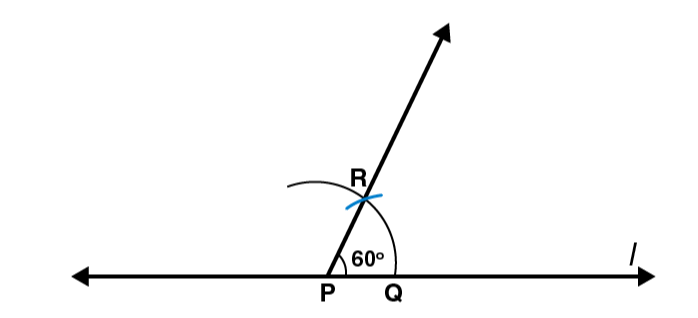(b) 300

To construct an angle of 300 the following steps can be followed

(i) Draw a line l and mark a point P on it. By Considering P as centre and with convenient radius, draw an arc of a circle such that it is intersecting the line l at Q.

(ii) Consider Q as centre and with the same radius as before, draw an arc intersecting the previously drawn arc at point R.

(iii) By Considering Q and R as centres and with radius more than 1 / 2 RQ draw arcs such that they are intersecting each other at S. Join PS which is the required ray making 300 with the line l.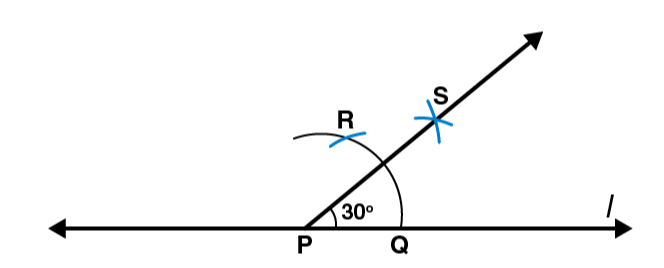(c) 900

To construct an angle of measure 900the following steps can be followed

(i) Draw a line l and mark a point P on it. Consider P as centre and with convenient radius, draw an arc of a circle such that it is intersecting the line l at Q.

(ii) Consider Q as centre and with the same radius as before, draw an arc intersecting the previously drawn arc at R

(iii) By Considering R as centre and with the same radius as before, draw an arc intersecting the arc at S as shown in figure

(iv) Now Consider R and S as centre, draw arc of same radius to intersect each other at T.

(v) Join PT, which is the required ray making 900 with the line l.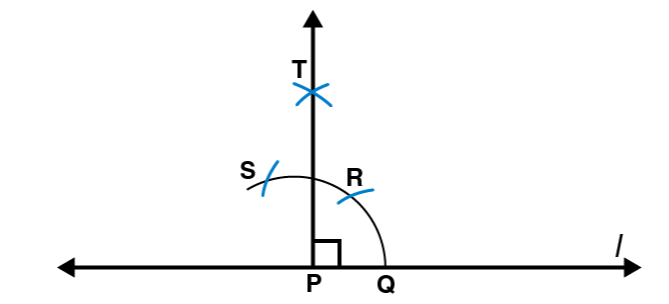(d) 1200

To construct an angle of measure 1200 the following steps can be followed

(i) Draw a line l and mark a point P on it. Considering P as centre and with convenient radius, draw an arc of circle such that it is intersecting the line l at Q.

(ii) By Considering Q as centre and with the same radius as before, draw an arc intersecting the previously drawn arc at R.

(iii) Consider R as centre and with the same radius as before, draw an arc such that it is intersecting the arc at S as shown in figure.

(iv) Join PS, which is the required ray making 1200 with the line l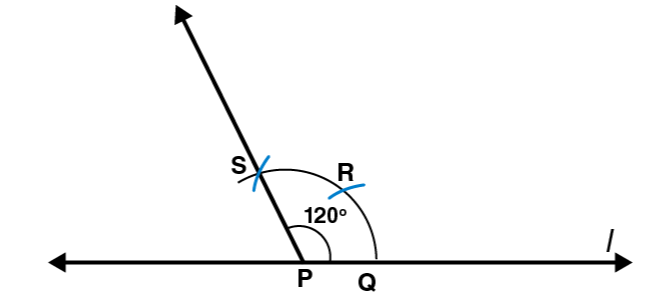(e) 450

To construct an angle of measure 450the following steps can be followed

(i) Draw a line l and mark a point P on it. Consider P as centre and with convenient radius, draw an arc of a circle such that it is intersecting the line l at Q.

(ii) Consider Q as centre and with the same radius as before, draw an arc intersecting the previously drawn arc at R

(iii) By Considering R as centre and with the same radius as before, draw an arc such that it is intersecting the arc at S as shown in figure.

(iv) Consider R and S as centres and draw arcs of same radius such that they are intersecting each other at T

(v) Join PT. Let this intersect the major arc at point U.

(vi) Now Consider Q and U as centres and draw arcs with radius more than 1 / 2 QU to intersect each other at point V. Join PV.

PV is the required ray making 450 with the line l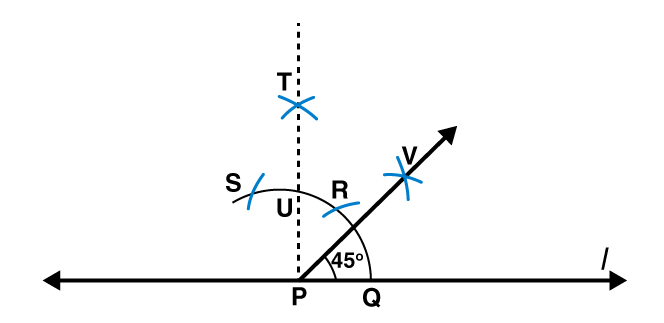(f) 1350

To construct an angle of measure 1350 the following steps can be followed

(i) Draw a line l and mark a point P on it. Considering P as centre and with convenient radius, draw a semicircle which intersects the line l at Q and R respectively.

(ii) By Considering R as centre and with the same radius as before, draw an arc intersecting the previously drawn arc at S

(iii) Considering S as centre and with the same radius as before, draw an arc such that it is intersecting the arc at T as shown in figure

(iv) Consider S and T as centres, draw arcs of same radius to intersect each other at U.

(v) Join PU. Let this intersect the arc at V. Now Consider Q and V as centres and with radius more than 1 / 2 QV, draw arcs to intersects each other at W.

(vi) Join PW which is the required ray making 1350 with the line l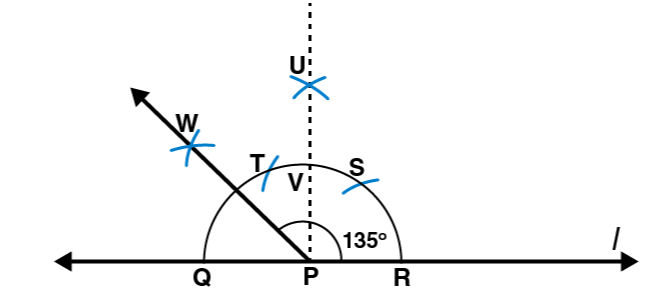(0)(0)